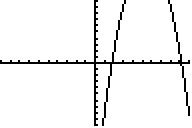# Knowledge Base

## Solution 29130: Calculating and Graphing Quadratic Regressions Using the TI-73 Family Calculator.

### How do I calculate and graph a quadratic regression using the TI-73 family calculator?

To calculate and graph quadratic regressions using the TI-73 family calculator, follow the example below.

Please Note: Texas Instruments recommends all TI-73 family users update the calculator to the latest operating system. More information on the latest operating system can be found at the TI-73 Explorer™ latest software website.

Example Data:

L1          L2
4 15
6 17
3 9
5 16

1) Press [2nd] [MEM]  [ENTER] to clear previous data stored in lists.
2) To begin inputting data, press the [LIST] key.
3) Input the example data in the L1 and L2 lists.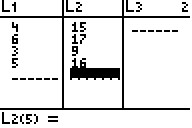5) Press [2nd] [STAT], scroll over to "CALC", then press  to select the "QuadReg" function. This will paste QuadReg on the home screen.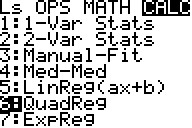6) Press [2nd] [STAT]  to input the L1 list.
7) Press the [,] key.
8) Press [2nd] [STAT]  to input the L2 list.
9) Press the [,] key.
10) Press [2nd] [VARS]   to select the "Y-Vars" menu and input the Y1 function.
11) Press [ENTER] to calculate the quadratic regression. This will also copy the regression equation to the Y= Editor so that the function can be graphed.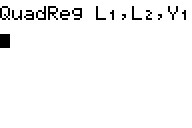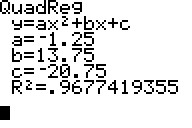The calculator should display values for the variables a, b, and c. Since the function for the quadratic regression is ax^2 + bx + c, the solution can be interpreted as:

-1.25x^2 + 13.75x - 20.75

Please Note: To display the correlation coefficient (R^2), execute the "DiagnosticOn" function by pressing [2nd] [CATALOG], scrolling down to DiagnosticOn and pressing [ENTER] ENTER].

12) Finally, to graph the quadratic regression function that is now stored to Y1, perform a "ZoomStat" by pressing [ZOOM] .